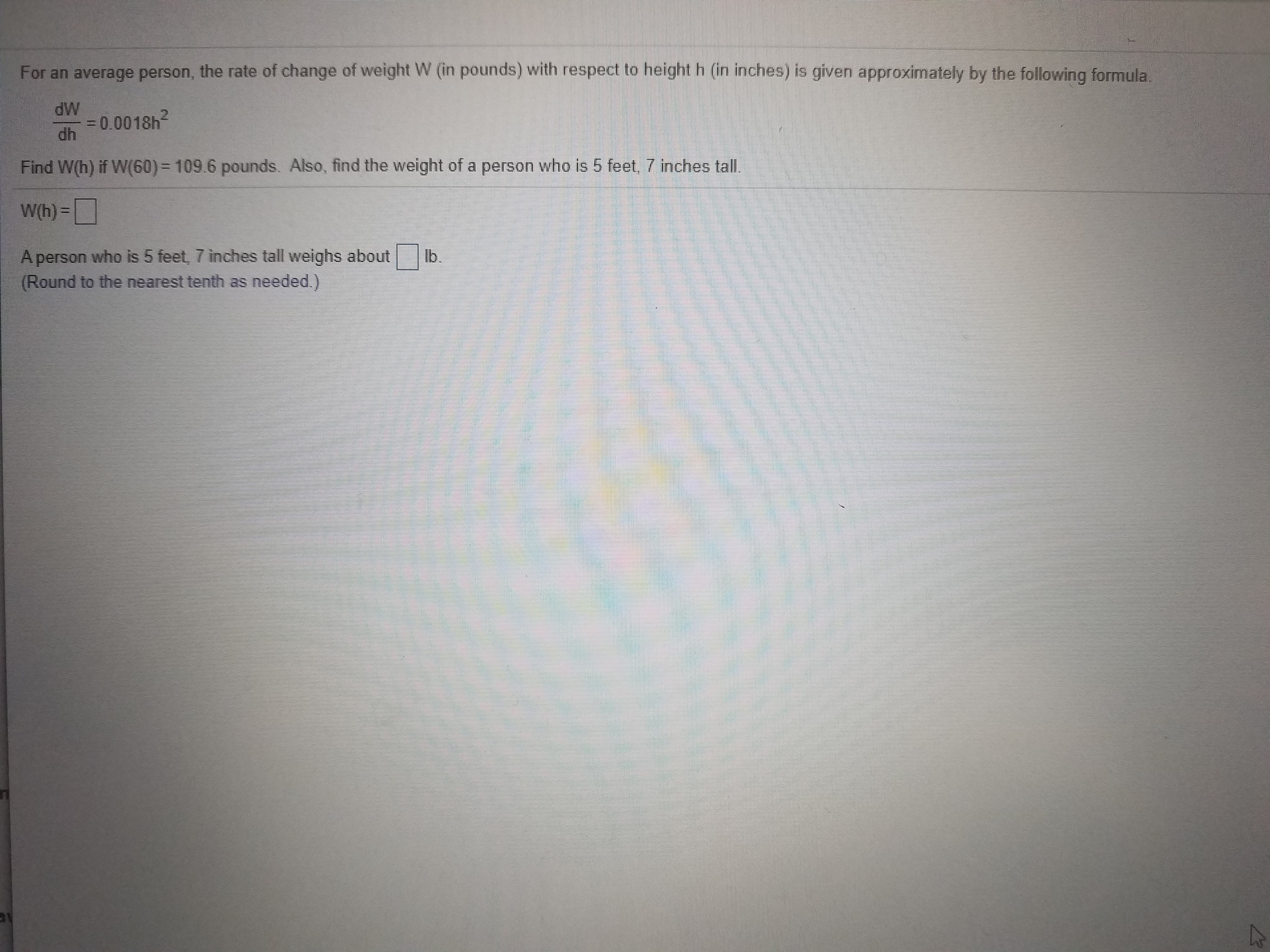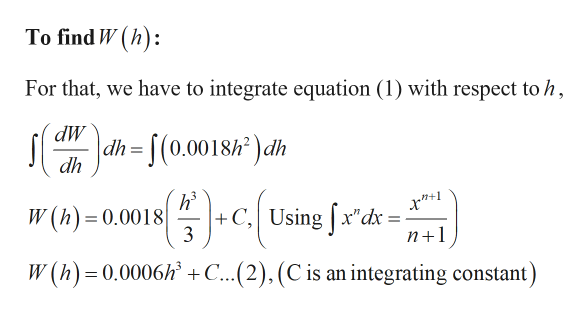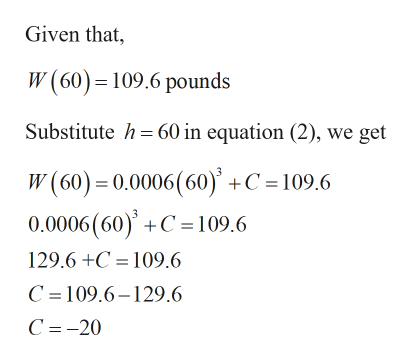# For an average person, the rate of change of weight W (in pounds) with respect to height h (in inches) is given approximately by the following formula,dW= 0.0018h?dh2.Find W(h) if W(60)= 109.6 pounds. Also, find the weight of a person who is 5 feet. 7 inches tallW(h) =|lb.A person who is 5 feet, 7 inches tall weighs about(Round to the nearest tenth as needed.

Question
15 viewshelp_outlineImage TranscriptioncloseFor an average person, the rate of change of weight W (in pounds) with respect to height h (in inches) is given approximately by the following formula, dW = 0.0018h? dh 2. Find W(h) if W(60)= 109.6 pounds. Also, find the weight of a person who is 5 feet. 7 inches tall W(h) =| lb. A person who is 5 feet, 7 inches tall weighs about (Round to the nearest tenth as needed. fullscreen
check_circle

Step 1

Given:

Step 2

Calculation:help_outlineImage TranscriptioncloseTo find W (h): For that, we have to integrate equation (1) with respect to h, dh= [(0.0018h² ) dh +C, Using [x"dx 3 fx'dx W (h) = 0.0018 %3D n+1 W (h) = 0.0006h +C...(2),(C is an integrating constant) fullscreen
Step 3help_outlineImage TranscriptioncloseGiven that, W (60) =109.6 pounds Substitute h = 60 in equation (2), we get W (60) = 0.0006(60)' +C =109.6 0.0006 (60) +C =109.6 129.6 +C = 109.6 C =109.6–129.6 C =-20 fullscreen

### Want to see the full answer?

See Solution

#### Want to see this answer and more?

Solutions are written by subject experts who are available 24/7. Questions are typically answered within 1 hour.*

See Solution
*Response times may vary by subject and question.
Tagged in

### Other